### Interpretation

For Moran’scoefficient,
indicates positive autocorrelation. Positive autocorrelation suggests that neighboring values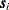and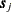tend to have similar feature valuesand, respectively. When
, this is a sign of negative autocorrelation, or dissimilar values at neighboring locations. A measure of strength of the autocorrelation is the size of the absolute difference
.

Geary’scoefficient interpretation is analogous to that of Moran’s. The only difference is that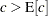indicates negative autocorrelation and dissimilarity, whereassignifies positive autocorrelation and similarity of values.

The VARIOGRAM procedure uses the mathematical definitions in the preceding section to provide the observed and expected values, and the standard deviation of the autocorrelation coefficients in the autocorrelation statistics table. The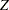scores for each type of statistics are computed as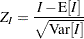for Moran’scoefficient, and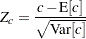for Geary’scoefficient. PROC VARIOGRAM also reports the two-sided p-value for each coefficient under the null hypothesis that the sample values are not autocorrelated. Smaller p-values correspond to stronger autocorrelation for both theandstatistics. However, the p-value does not tell you whether the autocorrelation is positive or negative. Based on the preceding remarks, you have positive autocorrelation when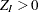or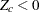, and you have negative autocorrelation whenor.